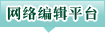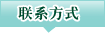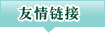E-Mail：

CN CN：11-1885/UTime-domain simulations of the submerged body's curvilinear motion near the water surface

DOI：

2020,42(4): 18-24 收稿日期：2018-11-29
DOI：10.3404/j.issn.1672-7649.2020.04.004

 韩端锋, 黄德波. 近水面潜体兴波阻力的数值预报和收敛性分析[J]. 船舶力学, 2005, 9(1): 29–35
HAN Duan-feng, HUANG De-bo. Numerical prediction and convergence analysis of wave resistance for submerged body near free surface[J]. Journal of Ship Mechanics, 2005, 9(1): 29–35
 杨向晖, 叶恒奎, 刘娟, 等. 潜体近水面航行兴波阻力计算[J]. 华中科技大学学报(自然科学版), 2008, 36(4): 129–132
YANG Xiang-hui, YE Heng-kui, LIU Juan, et al. Computation of the wave making resistance of submerged body moving beneath water surface[J]. J. Huazhong Univ. of Sci. & Tech. (Natural Science Edition), 2008, 36(4): 129–132
 ROKHLIN V. Rapid solution of integral equations of classical potential theory[J]. Journal of Computational Physics, 1985, 60(2): 187–207
 GREENGARD L, ROKHLIN V. A fast algorithm for particle simulations[J]. Journal of Computational Physics, 1987, 73(2): 325–348
 HACKBUSCH W, NOWAK Z P. On the fast matrix multiplication in the boundary element method by panel clustering[J]. Numerische Mathematik, 1989, 54(4): 463–491
 CHENG H, GREENGARD L, ROKHLIN V. A fast adaptive multipole algorithm in three dimensions[J]. Journal of Computational Physics, 1999, 155(2): 468–498
 LIN Z, LIAO S. Calculation of added mass coefficients of 3D complicated underwater bodies by FMBEM[J]. Communications in Nonlinear Science and Numerical Simulation, 2011, 16(1): 187–194
 CHEN Z S, WAUBKE H, KREUZER W. A formulation of the fast multipole boundary element method (FMBEM) for acoustic radiation and scattering from three-dimensional structures[J]. Journal of Computational Acoustics, 2008, 16(02): 303–320
 WANG Y, WANG Q, WANG G, et al. An adaptive dual-information FMBEM for 3D elasticity and its GPU implementation[J]. Engineering Analysis with Boundary Elements, 2013, 37(2): 236–249
 LIN Z, LIAO S. Calculation of added mass coefficients of 3D complicated underwater bodies by FMBEM[J]. Communications in Nonlinear Science & Numerical Simulation, 2011, 16(1): 187–194
 沈王刚, 郑尧坤, 林志良. 多极子面元法近水面椭球体兴波时域研究[J]. 舰船科学技术, 2018(8): 14–22
SHEN Wang-gang, ZHENG Yao-kun, LIN Zhi-liang. Multipole panel method for the time-domain wave making research for ellipsoid near free surface[J]. Ship Science and Technology, 2018(8): 14–22
 LIU Y J, NISHIMURA N. The fast multipole boundary element method for potential problems: a tutorial[J]. Engineering Analysis with Boundary Elements, 2006, 30(5): 371–381
 NISHIMURA N. Fast multipole accelerated boundary integral equation methods[J]. Applied Mechanics Reviews, 2002, 55(4): 299–324
 YOSHIDA K. Applications of fast multipole method to boundary integral equation method[J]. Kyoto: Kyoto University, 2001
 林志良. 比例边界有限元法及快速多极子边界元法的研究与应用[D]. 上海: 上海交通大学, 2010.
LIN Zhi-liang. Research and Application of Scaled Boundary FEM and Fast Multipole BEM[D]. Shanghai: Shanghaijiaotong University, 2010.

 读者ID： 密码：
 我要评论：